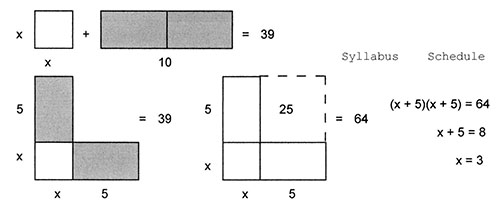####alt="schedule">

 Math 40 – Intermediate Algebra   Syllabus Fall ‘17 Section 3314 8-9:20 Rm Soc12 on M/W and Math 3 on T/R Instructor: Geoff Hagopian Office: Math 12 Phone: 776-7223 email: ghagopian@collegeofthedesert.edu Website: http://geofhagopian.net Office Hours: MTWR: 9:30-10:40

### Required Materials:

1.     Intermediate Algebra, a Modeling Approach  by Katherine Yoshiwara,
XYZ Textbooks, either the paperback or ebook version,
ISBN: 978-1-936368-34-1. Here is a hyperlink:
Here is a nice "getting started" guide:
http://www.xyzhomework.com/media/xyzhomework_getting_started_guide_student.pdf

2.   A scientific calculator is required for chapters 6 and 7. You will need the ability to compute logarithms, so look for a “log” and “lnkey.

### On-Line Assessment for Lab and Homework:

We will use the xyzhomework system.  To get started, you will need the xyzhomework ID for this course:

• Course xyzhomework ID: 13139

and follow the instructions for registering, logging in and enrolling in this course.

### In-Class Quizzes:

There will be regular in-class quizzes on the material we’re studying.

### Chapter Tests:

There will be 7 chapter tests (about 1 every 2 or 3 weeks -  see the schedule) plus a comprehensive final exam.

### The Math/Science Study Center in Room Math 4 and the ASC:

There are excellent tutoring services available in the Math and Science Study Center (Math 4) and in the Academic Skills Center, both of which are conveniently located in the immediate vicinity of our classrooms.

### Course Summary:

 Category Weighted % Homework Assignments 20% Chapter Tests 60% Final Exam 15% In-class quizzes 5% Total 100%

Students completing this course are generally understood to be able to
1. Demonstrate number sense, which is characterized by the ability to judge relative sizes of numbers, perform computations with numbers in different representations, represent sets of numbers using diagrams, and assess the reasonableness of results.
2. Generalize arithmetic operations by using the concept of variable to include change and patterns.
3. Use the information obtained in application problems to estimate a reasonable solution, identify and execute methods of a solution that involve algebraic computations, and evaluate the reasonableness of results.
4. Apply algebraic principles and deductive reasoning to solve equations and inequalities.
5. Describe functional relationships numerically, symbolically, verbally, and graphically. Not necessarily in that order.